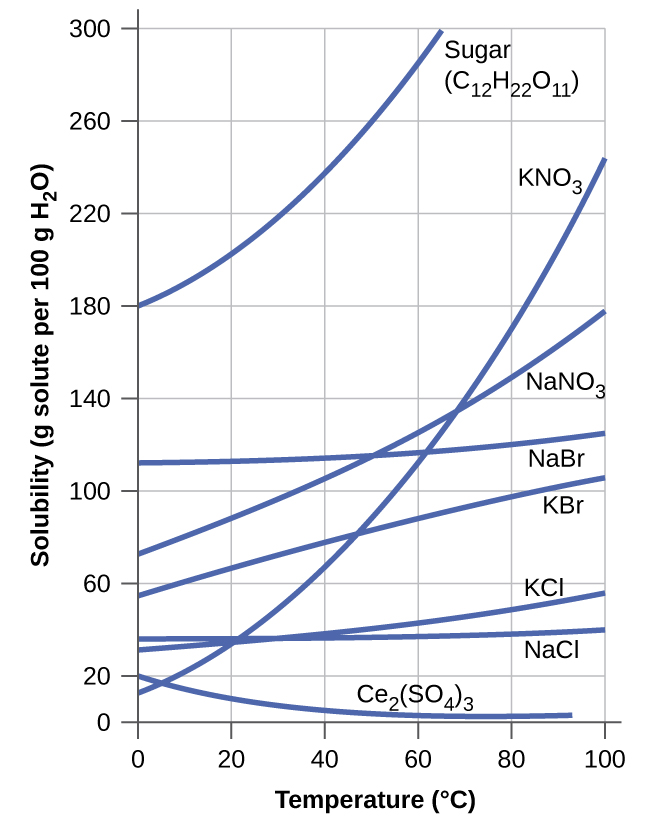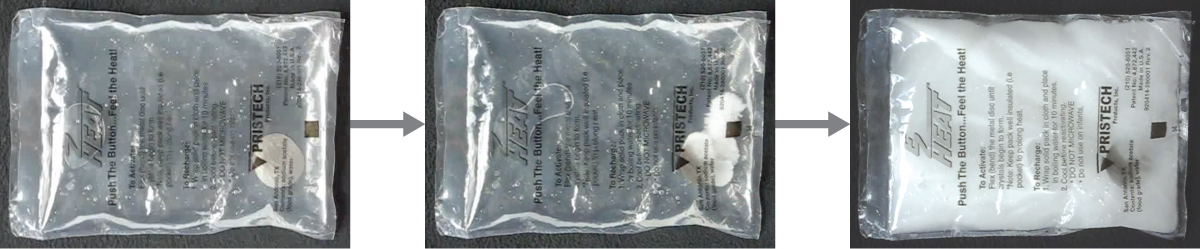# 11.3 Solubility  (Page 5/13)

 Page 5 / 13

## Solutions of solids in liquids

The dependence of solubility on temperature for a number of inorganic solids in water is shown by the solubility curves in [link] . Reviewing these data indicate a general trend of increasing solubility with temperature, although there are exceptions, as illustrated by the ionic compound cerium sulfate.This graph shows how the solubility of several solids changes with temperature.

The temperature dependence of solubility can be exploited to prepare supersaturated solutions of certain compounds. A solution may be saturated with the compound at an elevated temperature (where the solute is more soluble) and subsequently cooled to a lower temperature without precipitating the solute. The resultant solution contains solute at a concentration greater than its equilibrium solubility at the lower temperature (i.e., it is supersaturated) and is relatively stable. Precipitation of the excess solute can be initiated by adding a seed crystal (see the video in the Link to Learning earlier in this module) or by mechanically agitating the solution. Some hand warmers, such as the one pictured in [link] , take advantage of this behavior.This hand warmer produces heat when the sodium acetate in a supersaturated solution precipitates. Precipitation of the solute is initiated by a mechanical shockwave generated when the flexible metal disk within the solution is “clicked.” (credit: modification of work by “Velela”/Wikimedia Commons)

## Key concepts and summary

The extent to which one substance will dissolve in another is determined by several factors, including the types and relative strengths of intermolecular attractive forces that may exist between the substances’ atoms, ions, or molecules. This tendency to dissolve is quantified as substance’s solubility, its maximum concentration in a solution at equilibrium under specified conditions. A saturated solution contains solute at a concentration equal to its solubility. A supersaturated solution is one in which a solute’s concentration exceeds its solubility—a nonequilibrium (unstable) condition that will result in solute precipitation when the solution is appropriately perturbed. Miscible liquids are soluble in all proportions, and immiscible liquids exhibit very low mutual solubility. Solubilities for gaseous solutes decrease with increasing temperature, while those for most, but not all, solid solutes increase with temperature. The concentration of a gaseous solute in a solution is proportional to the partial pressure of the gas to which the solution is exposed, a relation known as Henry’s law.

## Key equations

• ${C}_{\text{g}}=k{P}_{\text{g}}$

## Chemistry end of chapter exercises

Suppose you are presented with a clear solution of sodium thiosulfate, Na 2 S 2 O 3 . How could you determine whether the solution is unsaturated, saturated, or supersaturated?

Supersaturated solutions of most solids in water are prepared by cooling saturated solutions. Supersaturated solutions of most gases in water are prepared by heating saturated solutions. Explain the reasons for the difference in the two procedures.

The solubility of solids usually decreases upon cooling a solution, while the solubility of gases usually decreases upon heating.

Suggest an explanation for the observations that ethanol, C 2 H 5 OH, is completely miscible with water and that ethanethiol, C 2 H 5 SH, is soluble only to the extent of 1.5 g per 100 mL of water.

Calculate the percent by mass of KBr in a saturated solution of KBr in water at 10 °C. See [link] for useful data, and report the computed percentage to one significant digit.

40%

Which of the following gases is expected to be most soluble in water? Explain your reasoning.

(a) CH 4

(b) CCl 4

(c) CHCl 3

At 0 °C and 1.00 atm, as much as 0.70 g of O 2 can dissolve in 1 L of water. At 0 °C and 4.00 atm, how many grams of O 2 dissolve in 1 L of water?

2.80 g

(a) How did the concentration of dissolved CO 2 in the beverage change when the bottle was opened?

(b) What caused this change?

(c) Is the beverage unsaturated, saturated, or supersaturated with CO 2 ?

The Henry’s law constant for CO 2 is 3.4 $×$ 10 −2 M /atm at 25 °C. What pressure of carbon dioxide is needed to maintain a CO 2 concentration of 0.10 M in a can of lemon-lime soda?

2.9 atm

The Henry’s law constant for O 2 is 1.3 $×$ 10 −3 M /atm at 25 °C. What mass of oxygen would be dissolved in a 40-L aquarium at 25 °C, assuming an atmospheric pressure of 1.00 atm, and that the partial pressure of O 2 is 0.21 atm?

How many liters of HCl gas, measured at 30.0 °C and 745 torr, are required to prepare 1.25 L of a 3.20- M solution of hydrochloric acid?

102 L HCl

what are oxidation numbers
pls what is electrolysis
Electrolysis is the process by which ionic substances are decomposed (broken down) into simpler substances when an electric current is passed through them. ... Electricity is the flow of electrons or ions. For electrolysis to work, the compound must contain ions.
AZEEZ
thanks
Idowu
what is the basicity of an atom
basicity is the number of replaceable Hydrogen atoms in a Molecule. in H2SO4, the basicity is 2. in Hcl, the basicity is 1
Inemesit
how to solve oxidation number
mention some examples of ester
do you mean ether?
Megan
what do converging lines on a mass Spectra represent
would I do to help me know this topic ?
Bulus
oi
Amargo
what the physic?
who is albert heistein?
Bassidi
similarities between elements in the same group and period
what is the ratio of hydrogen to oxulygen in carbohydrates
bunubyyvyhinuvgtvbjnjnygtcrc
yvcrzezalakhhehuzhbshsunakakoaak
what is poh and ph
please what is the chemical configuration of sodium
Sharon
2.8.1
david
1s²2s²2p⁶3s¹
Haile
2, 6, 2, 1
Salman
1s2, 2s2, 2px2, 2py2, 2pz2, 3s1
Justice
1s2,2s2,2py2,2
Maryify
1s2,2s2,2p6,
Francis
1s2,2s2,2px2,2py2,2pz2,3s1
Nnyila
what is criteria purity
cathode is a negative ion why is it that u said is negative
cathode is a negative electrode while cation is a positive ion. cation move towards cathode plate.
king
CH3COOH +NaOH ,complete the equation
compare and contrast the electrical conductivity of HCl and CH3cooH
The must be in dissolved in water (aqueous). Electrical conductivity is measured in Siemens (s). HCl (aq) has higher conductivity, as it fully ionises (small portion of CH3COOH (aq) ionises) when dissolved in water. Thus, more free ions to carry charge.
Abdelkarim
HCl being an strong acid will fully ionize in water thus producing more mobile ions for electrical conduction than the carboxylic acid
Valentine
differiante between a weak and a strong acid
david
how can I tell when an acid is weak or Strong
Amarachi
an aqueous solution of copper sulphate was electrolysed between graphite electrodes. state what was observed at the cathode
write the equation for the reaction that took place at the anode
Bakanya
what is enthalpy of combustion
Bakanya
Enthalpy change of combustion: It is the enthalpy change when 1 mole of substance is combusted with excess oxygen under standard conditions. Elements are in their standard states. Conditions: pressure = 1 atm Temperature =25°C
Abdelkarim
Observation at Cathode: Cu metal deposit (pink/red solid).
Abdelkarim
Equation at Anode: (SO4)^2- + 4H^+ + 2e^- __> SO2 + 2H2O
Abdelkarim
Equation : CuSO4 -> Cu^2+ + SO4^2- equation at katode: 2Cu^2+ + 4e -> 2Cu equation at anode: 2H2O -> 4H+ + O2 +4e at the anode which reacts is water because SO4 ^ 2- cannot be electrolyzed in the anode
NikenByByBy Jazzycazz JacksonBy OpenStaxBy OpenStaxBy OpenStaxBy Steve GibbsBy Madison ChristianBy OpenStaxBy Jonathan LongBy Royalle MooreBy OpenStax